# Bayesian Musings from a Non-Statistician

## Ah-ha! moments in making sense of Bayesian statistics

This post is aimed at those who have already dipped their toe into Bayesian statistics without any rigorous formal training i.e. myself.

• 101 Recap

# 101 Recap

1. “Probability and statistical inference have inverse objectives”

With probability modelling we set the parameters for a specified model and simulate potential outcomes. Statistical inference on the other hand ingests measurements from the world and backtracks to estimate the parameters of a chosen model.

The bidirectional relationship underpins the way we validate statistical techniques’ potential to capture the essence of reality: we synthesise data from a known distribution, blur the signal with some noise that represents random sampling error and estimate the true parameters, point estimates.

Similarly, Bayesians criticise and revise their models by analysing a posterior predictive distribution (c.f point estimates from before). These “posterior predictive checks” examine the deviation of data simulated by the fitted posterior model from the observed data.

2. “Conditional probabilities can be visualised”

Conditional probability has nothing to do with Bayesian vs frequentist inference. It represents a recalculation of probability that accounts for additional information on which the event of interest depends (i.e. if not independent). One way to visualise conditional probabilities is as areas within a complete probability space. The event being conditioned on collapses the sample space from the “universe” to that particular event.

Hypotheses are uncertain descriptions of what the world looks like. They can can either be discrete (innocence or guilt, which die did I roll) or continuous (mean level of gene expression). Bayesians in either case think of stretching a probability distribution across the hypotheses (for both prior and posterior). There is nothing fundamentally different between the prior and the posterior — they represent your state of knowledge at any point in the process with the data you’ve observed so far.

More specifically we throw data into the likelihood (by initialising the random variable to these fixed observed values) so that it “tugs” on the prior distribution of each of the parameters for that likelihood parameters (both functions of the unknown parameters, see Appendix A).

# Primary Frequentist Differences

1. “Statistics is the science of changing your mind”

Frequentist statistics is characterised by changing your mind about default actions based on the “unsurprising-ness” of the data (the [in]famous p-value). Bayesians change their minds about prior beliefs instead. Frequentists need not form a belief to have a default action — this is purely something one commits to without analysing any data.

2. “Source of uncertainty”

Inference from a frequentist’s perspective says that sampled data is drawn from a large or infinite sample space that we may take expectations over. The randomness of data gives the standard error in regression coefficient estimates — uncertainty in what would happen to the estimates if we observed more data sets that look like our own. This sampling error is the variance in a classifier’s predictions as discussed in more explicit machine learning contexts (linear regression is a part of the ML toolkit after all). A high variance means you’ve overfit on your one sample and have claimed to make sense out of the randomness present.

Bayesians reason instead that a probability distribution represents the unknown-ness of a quantity, not the randomness of the quantity from the data. The uncertainty in one’s state of knowledge of the system is described by their strength of “belief” about the true and unknown parameter values for the observed data thus far.

Being pedantic, while the source of uncertainty is different, Bayesians may agree with frequentists that the “true” parameter takes a fixed value that we would like to know, whether fixed from the start or potentially generated from a physically random mechanism. There are however instances in Physics, say the uncertainty principle, where we nature fundamentally can only ever reveal a distribution.

Since frequentists don’t regard the unknown parameter as a random variable, P(H0|D) makes no sense in classical hypothesis testing; the truth of a particular hypothesis is not a random variable. It is either true or it’s not. This is one of the mistakes in suggesting that a p-value is the “probability that an observation is due to chance” when it is computed assuming H0 is true, that sample differences are caused random chance. The p-value is instead P(D*|H0) where D* refers to the observed, or more extreme, data.

Either way the frequency guarantees of confidence intervals and uncertainty quantification of credible intervals converge once there is enough information.

# Some Ah-ha!s

1. “Bayes’ theorem Bayesian inference. The first is a general formula, the second is statistical”

Bayes’ theorem is not a Bayesian approach per se. The theorem is a formula foundational to probability theory that connects inverse conditional probabilities. It allows us to invert relative frequencies derived from contingency tables just fine (e.g. a confusion matrix).

What makes one a Bayesian is quantifying uncertainty with probability over hypotheses

Bayesian inference is the quantitative method to update ones’ beliefs given new information that manifests as a reallocation of probability across hypotheses.

It’s worth prefacing that only in the most trivial cases do data have deterministic relations to candidate causes; where conditional probability tables contain 0s and/or 1s (e.g. a 3, 6 and 8 sided dice are in a box and rolling an unknown dice to give a 7 rules out the first two hypotheses). In reality inferences are probabilistic and the data relate to their underlying causes with degrees of certainty (as well as being subject to measurement noise).

Nonetheless, if we have complete knowledge of the probability space of mutually exclusive hypotheses — we know exacts priors (base rates) and likelihoods for all hypotheses — then Bayes’ formula computes the posterior exactly. Such is the case when we roll a die that is randomly selected from a box whose contents we already know, or we have complete population information in a contingency table. This is deductive logic of probability theory, and it gives a direct way to compare hypotheses, draw conclusions, and make decisions. The Monty Hall problem presents another such case.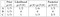Monty Hall Problem: complete knowledge of the prior and posterior probability spaces. You select door A, H is the hypothesis a prize is behind door {A,B,C}, and D represents the event that Monty opens door B and no prize is there. Bayes’ theorem makes the complexity of the problem more manageable and computes full probabilities, although a maximum likelihood approach will also tell you to switch

A frequentist would have no problem with this and gets to the same answer using relative frequencies, as can be demonstrated with hard code:

However, in most experiments the prior probabilities of hypotheses are not known exactly. When this is the case, our recourse is the art of statistical inference. We either introduce a prior (Bayesian treatment) or do our best using only the likelihood (frequentist treatment). Note that if we estimate our probabilities with relative sample frequencies and plug them into Bayes’ formula this is still not true Bayesian inference since it produces a point estimate.

2. “How Bayesian reasoning can give us both “correct” but also “subjective” probabilities”

In the previous point we decoupled Bayes’ theorem from Bayesian inference. This point follows then quite naturally; we can access conditional probabilities of real interest using Bayes’ theorem that control for dependence between events. For example the canonical disease screening example highlight the correct conditional value we should be concerned with.Screening testing example: event A is actually having the disease, and event B testing positive for it. The prior P(A) is disease prevalence and we often have a lot of data on this. We have a test for cancer, separate from the event of being diagnosed with cancer. Tests are of course also flawed and so the the probability of a result one way or another is weaker than it might initially sound.

On the other hand the Bayesian interpretation of probability, as credibility specific to an individual with a certain state of knowledge, doesn’t sit well with many frequentists due to its “subjectivity” — should science accept that different individuals with identical data may sensibly reach different conclusions? (It can however be argued that the likelihood is also subjective — Bayesian modellers commit a priori to a joint distribution of the data and parameters, p(θ, data), and not simply the prior).

3. “Precision and Recall are directly related through Bayes theorem”

This becomes clear if we define precision and recall as conditional probabilities, for disease present D and positive test +ve.

Precision: P(D|+ve)
Recall: P(+ve|D)

Read from right to left, precision asks “given a +ve test, what’s the probability the disease is present” while recall asks “given a disease present, what’s the probability the test is +ve”. These are two very different things and wrong to assume that a screening test with high recall implies a high precision too — this is the prosecutor’s fallacy (explained visually next). One can have 100% recall but low precision due to false positives i.e. P(D|+ve) ≪ P(D|+ve). More realistically, the product of a small disease prevalence (large P(D’)) with a non-insignificant false alarm rate P(+ve|D’) can reduce the value of precision far below that of the recall.

4. “The effect of a small prior done visually” (the prosecutor’s fallacy)

A well-reasoned concern was published by a heart doctor on the new ECG feature on the Apple. The crux of his piece rested on the rarity of people with atrial fibrillation (about 1%) which would cause false alarms, and many false alarms given the number of sales one can expect from a company that generated more profit in 3 months than Amazon has ever done. The red line below left should be committed to memory by anyone who builds or uses classifiers or diagnostic tests; small priors have a dramatic effect on false positives even with tests that have a specificity = sensitivity = 99%!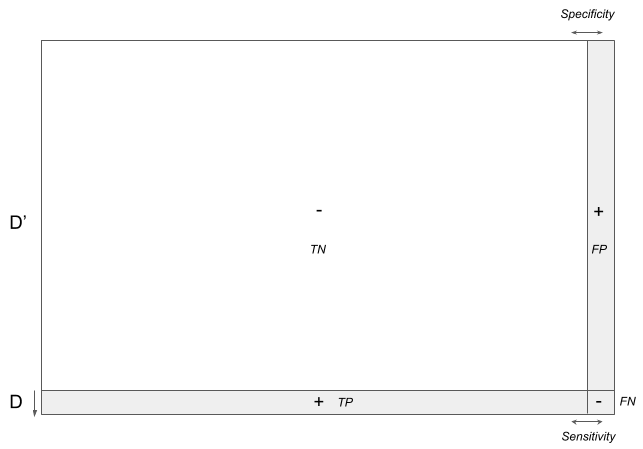Left, probability of a true disease given a positive test, with specificity = sensitivity = 99%, revealing dramatic variation with the magnitude of the prior. Right, grey areas are the quadrants needed to calculate P(D|+ve), which is underdetermined from the sensitivity and specificity of a screening.

One way to understand why this is is to consider a confusion matrix whose areas are proportional to a quadrant’s probability. If we artificially vary the prior D (normally a fixed estimate from the data) toward 0 we get a precision 1/(1+FP/TP) that tends to 0 as TP falls quickly due to its change in area whilst FP increases.

It’s worth pointing out that while precision (and NPV) are dependent on the population being tested — influenced by the prevalence of disease — sensitivity, P(+ve|D), and specificity, P(-ve|D’), are conditioned on the diseased/healthy state (they are determined horizontally above).

ML Tangent
Since recall and specificity are conditioned on the class label, ROC curves are invariant to the baseline probability (class imbalance) compared to precision-recall (PR) curves. They typically give an optimistic (deceptive?) picture of model performance on datasets with class imbalance (because of the use of true negatives in the False Positive Rate in the ROC Curve which PR curves carefully avoid).

Where baseline probabilities of the problem are important, and the “positive” class is more interesting than the negative class, PR curves may be more useful in characterising classifier performance.

Another way to see the effect of a small prior is by representing the probabilities as Venn diagrams which allow the fractions to be visualised more readily.

The graphic shows again how a rare disease (small red area) with a test that has 99% sensitivity/recall (large intersection within red) and 99% specificity (large white area) can still yield many false positives and hence low precision (large green area).

I’ve left one final intuitive tool, using natural frequencies, in Appendix B.

Empirical Bayes is useful in ranking and analysing a large list of proportions such as CTRs and gene expression levels. Knowledge of the entire distribution is brought into each estimate and shrinks the credible intervals whilst pulling the expected values toward it, particularly those observations with smaller amounts of data. It may be viewed as an approximation to a fully Bayesian treatment of a hierarchical model (see below) where the hyperpriors are set to their most likely values, instead of being integrated out.

6. “Prior information can formally inject common-sense into the equation”

In the contrived coin toss experiment with coin of bias p, the Bayesian’s input of common sense manifests as a “head start” in their maximum a posteriori estimate of the coin’s bias for a Beta distribution of potential biases,

with number of successes s and failures f. For a uniform prior, Beta(α=1,β=1), we revert to the intuitive maximum likelihood answer of s/(s+f) from the pure frequentist who assumes that the sample data is representative of the population of coin tosses because that’s all there is. This makes a difference with small sample sizes. “4 heads and 0 tails you say? The coin is 100% biased”.

However it should be said that since Bayesians have a full posterior distribution over parameter values there is a preference for expected values — integrating the full posterior to account for full uncertainty and potential multi-modality.

7. “Prior information can regularise a model”

Lasso regression constrains large coefficient estimates and shrinks some towards zero, effectively performing variable selection and choosing a simpler model. This avoids overfitting and is much better suited in dealing with datasets that contain collinearity.

Another way to phrase this is that we have a prior belief that most coefficients should be close to zero, and this can be expressed through a prior distribution (rather than through a cost function with a penalty term). This is exactly what can be achieved using Bayesian regression with the appropriate priors.

8. “Uncertainty in your neural network predictions”

Bayesian deep learning aims to move from point estimates of weights to full distributions for all latent variables. As a by-product we get to propagate through the uncertainty of random variables to the uncertainty of the prediction. Note that we effectively double the number of parameters we must learn (mean and spread).

Wide and deep neural networks represent more complex Bayesian models that can contain millions of parameters and in these instance Monte-Carlo methods are slow to converge — they may take weeks to discover the full posterior. To help solve this a technique called Variational Inference has been gaining momentum through probabilistic programming libraries such as Uber’s Pyro and Google’s Edward2.

# MCMC

1. “Monte Carlo techniques are not just for Bayesians”

What’s well known is the use of bootstrap resampling to compute the standard error in an arbitrary statistic using sample data without ever having seen the parent or population distribution. But what is less well known is that random sampling can be used to produce a much more flexible null hypothesis significance test (NHST) framework than an “off-the-shelf” analytical model.

With such a framework you have full choice over the test statistic and are forced to make your model assumptions explicit in setting up the simulations. It can be useful to prototype different test statistics and models that may turn out to yield different results — something potentially noteworthy. Packages such as infer do just that.

For example, if you wanted to compute the p-value of the Hamming similarity between two vectors you could:

1. Randomly shuffle your two vectors and compute the Hamming similarity (the null hypothesis is that the vectors are unrelated)

The proportion of times that the simulated vectors were more similar than the original vectors, x/N, is the (one-sided) p-value by definition.

2. “We still care about the normalising constant P(data) in sampling problems”

If we only care about point estimates in our posterior (e.g. find the MAP) we can drop P(data), a normalising constant, since we just care about exploring the parameter space to maximise likelihood * prior. This is an optimisation problem: find θ that maximises the proportionality. P(data) is equally irrelevant if doing hypothesis comparison on the same dataset using the Bayes Factor.

This situation is different for MCMC sampling problems. A denominator is necessary to squeeze out fully-fledged probabilities. This is a sampling distribution problem: find P(θ|data), which requires integration over the entire sample space. This can be computationally difficult!

3. “The intractability of the normalising constant”

Notice how marginalising out θ in the denominator is the same problem as attempting to normalise the numerator with an unknown constant so that it integrates to 1. This is by deliberate setup of a “probability” — from the law of total probability the numerator will be a subset of the denominator that defines the scope of the entire space.

Consider a system of binary variables (say quantum spin states in the Ising model) where we seek a probability mass function of the spin configurations. Calculating the integral in the denominator (where x is the data) can be difficult even here since to produce a normalised probability we must sum over all of the possible spin configurations which increases exponentially with the number of variables. For a small 10 x 10 grid this is 2¹⁰⁰ different configurations to calculate the average magnetisation.

MCMC is our heuristic knight in shining armour — it allows us to sample from a distribution g(x)/k without knowing or caring about k and it does this by stepping through the probability space with a weighted random walk that’s biased toward the more likely states.

# AB Testing

1. “Hypothesis testing = Parameter estimation”

To answer questions that are posed as hypothesis tests, we can elegantly transpose the question to be one of parameter estimation and inferring the HPD. This style feels much more natural and in this way we get the effect sizes between groups. The PyMC3 library uses deterministic random variables (values fully determined by their parent values) to handle all the transformations necessary under the hood that ultimately output the desired P(B-A > 0).

NHST (say a Chi-square test or logistic fit) can at most tell us “these two things are not likely to be the same” (unless we bake a difference threshold into our hypotheses).

When running a test we’re often aiming to not just understand whether a variant will perform better, but by how much. For example, if testing whether a drug will improve IQ more than a placebo, if we have achieved a boost of 3 IQ points, even within our posterior credibility interval, can we really say that the drug is effective and worthy of approval?

The primary focus of NHST is avoiding Type I errors; there is a preference to stick to default actions (the null hypothesis is assigned with this assumption). If a new recommendation algorithm is only slightly better than the current model but has a p-value of 0.11, we might be tempted to discard it. However not all false positives are created equally. For example, choosing variant B with CTR 8% and A is 8.3% is a very different mistake to make than if A were 15%. Again it’s the magnitude that is also of interest.

4. “Remind me, what’s a p-value again?”

Interpretability is crucial in communicating ideas and articulating byzantine concepts like p-values takes some care. Would you rather report to your boss that “the probability that A is greater than B is 7%”, or perhaps “assuming the null hypothesis that A is equal to B, the probability that we would see a result at least this extreme in A versus B is 3%”?

Bayesians are also better equipped to posit stronger statements to make decisions from e.g. P(B-A > δ) where δ can be any difference we specify (set δ=0 for any improvement).

# Hierarchical Modelling

1. “Assumption: observations are related to one another”

Hierarchical modelling can be seen as a middle-ground between pooling all measurements together and creating separate regressions for treatment groups. It is distinct from empirical Bayes since we are no longer estimating hyperpriors but are modelling their uncertainty through a hyperprior distribution.

Through such a design we impose a model structure with our assumptions that our treatment groups of observations are related to one another. Depending on the scenario this can be sensible, say justified using the biological concept of common descent. Everything we use to justify our model structure comes from assumptions extrinsic to the data — domain knowledgethat we still have to qualitatively and quantitatively verify. There is no free lunch; we have to describe our assumptions explicitly and encode them in the model structure or in the probability distributions used e.g. you might ultimately justify a parsimonious model following Occam’s razor.

“All models are wrong, but some are useful” — George E. P. Box

2. “Rare species are not alone”

When we have a new sample for which we have very few observations we are able to borrow evidence from the rest of the population to make more tightly-bound inferences about the new sample. In some cases the collection of new data is simply not feasible e.g the beak length of a new rare species of bird.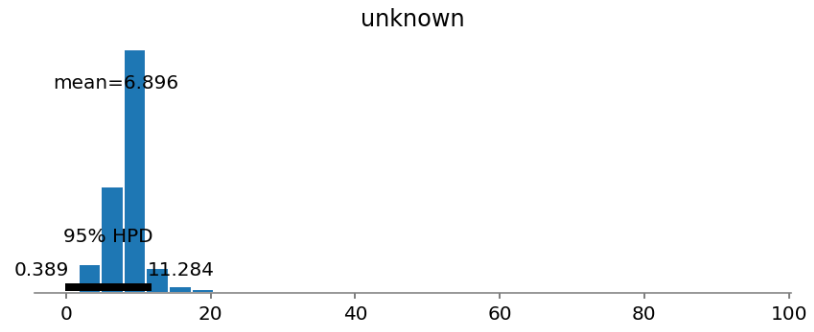Before (left) and after (right) applying a hierarchical design — we see “variance shrinkage” in our estimates of the unknown species’s beak depth.

A non-hierarchical design in this scenario will give a nonsensically wide credible interval (characterised by the high posterior density [HPD] interval) for a rare species. This is because we impose identical uninformative priors on each species that “let the data speak for itself” thus leaving us with large uncertainties. In the non-hierarchical case, the prior parameters are point estimates and not drawn from a parent distribution.

3. “The generative story” and “information pooling”

The generative story is as follows: the population as a whole has a parental hyperprior distribution that constrains the region from which the prior parameters of individual species are drawn (independently). Ultimately all the data influences estimates from global hyperprior distributions which in turn influence the individual estimates and this manifests with the aforementioned shrinkage effect. We could justify this assumption of underlying similarity in this case by deferring to common ancestry, or when inferring the bias of different coins produced by the same mint.

If the individual prior parameters are strongly dependent on the shared hyperprior (a strong correlation), the posterior of the smaller N species are strongly influenced by the data from the larger N species (see Appendix C).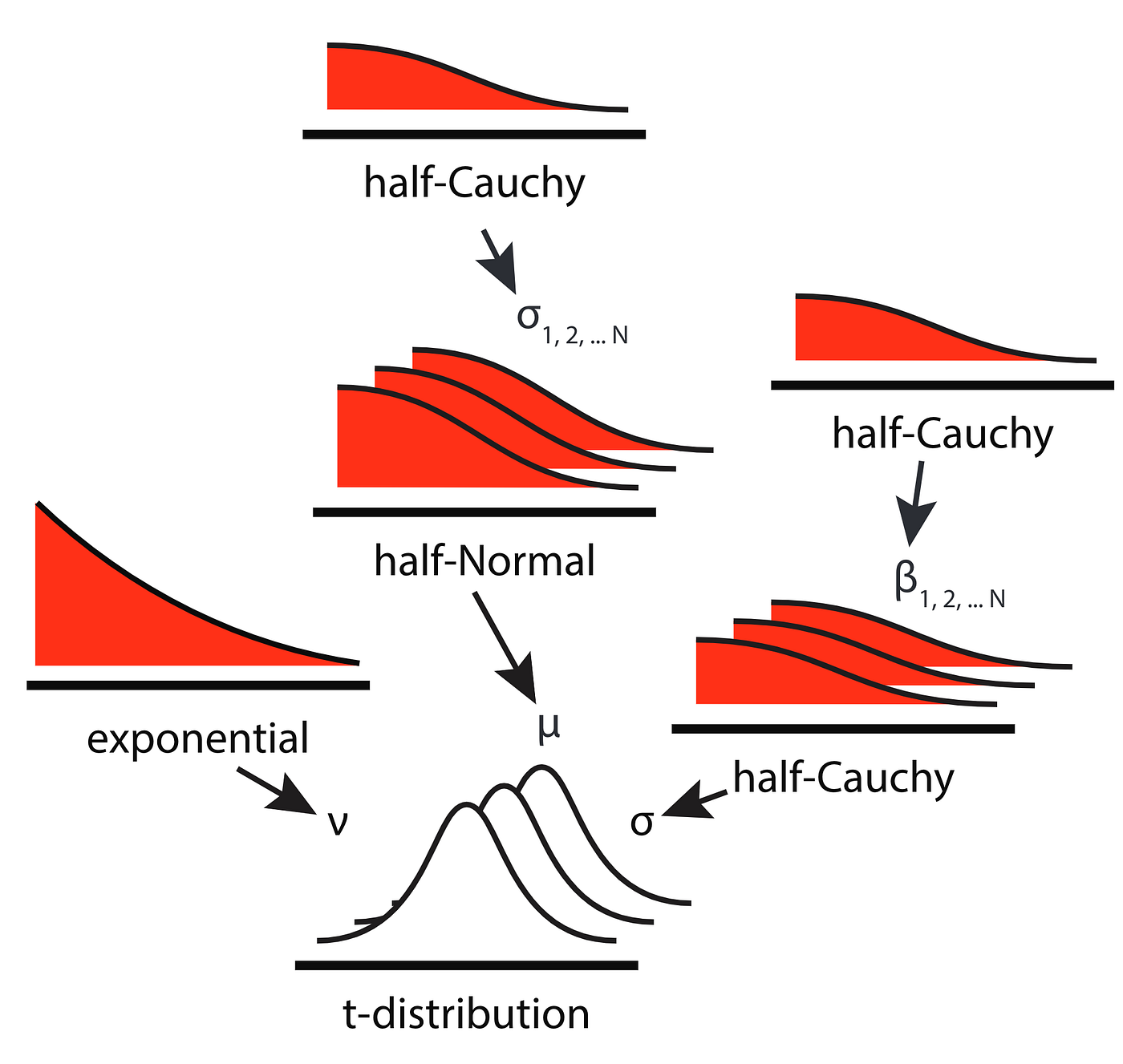The arrows represent dependencies and describe data generation where sampling the t-distribution P(y|σ,μ,𝜈) yields outcomes. The 3 layered distributions symbolise 3 individual species. For N species there are 2N+1 parameters estimated simultaneously here (𝜈 is global). Hyperpriors one level up are good enough here to capture the hierarchical structure. https://github.com/ericmjl/bayesian-stats-modelling-tutorial

If we were to simplify the above chain of dependencies such that only μ depended on a hyperprior (i.e. take the central chain only) for each species we would get the joint posterior:

P(σ,μ|y) ∝ P(y|σ,μ)P(σ,μ) = P(y|μ)P(μ|σ)P(σ)

• By the definition of a hierarchical model we have a factorised chain of dependencies, one for each distribution in the chain

# Appendix

A.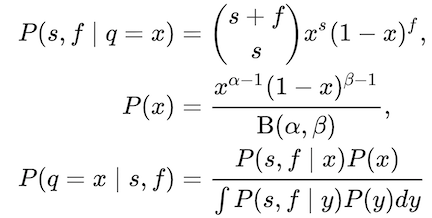A binomial likelihood distribution with its Beta conjugate prior, both viewed as functions of the parameter x (the probability of success). Empirical data of s successes and f failures is fed in and a distribution of the random variable q is cranked out.

B.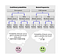Both calculations are forms of Bayes’s theorem. Natural frequencies can make it easier to infer precision and more intuitive to consider the effects of a small prior. It is clearer here the at the product of a small prior and and a non-insignificant false alarm rate reduces the precision.

C.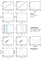Taken from “Bayesian Data Analysis” by Kruschke. Notice the similarity for the two different (coin bias) parameters in the posterior distributions in the bottom two graphs that result from the strong dependence between the individual bias parameters (thetas) and their parental hyperprior, in the top two left graphs